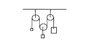# 3 mass, 3 pulley

jkw

## Homework Statement

--------------
I I I = string
O O O = massless frictionless pulley
I II I
' O " . = mass 1
: : = mass 2
" = mass 3

Find acceleration of each mass.

## Homework Equations

F=ma
conservation of String

## The Attempt at a Solution

Tension is same
T-m1g = m1a1
2T-m2g = m2a2
T-m3g = m3a3

a1=a3=1/2a2 <- I am not sure how to use conservation of string... shouldn't a1 is equal to a3 because there are connected to same sting..
so my question is how to get relationship between/among accelerations

#### Attachments

•q.jpg
6.6 KB · Views: 357
Last edited:

azizlwl

## Homework Statement

--------------
I I I = string
O O O = massless frictionless pulley
I II I
' O " . = mass 1
: : = mass 2
" = mass 3

Find acceleration of each mass.

## Homework Equations

F=ma
conservation of String

## The Attempt at a Solution

Tension is same
T-m1g = m1a1
2T-m2g = m2a2
T-m3g = m3a3

a1=a3=1/2a2 <- I am not sure how to use conservation of string... shouldn't a1 is equal to a3 because there are connected to same sting..
so my question is how to get relationship between/among accelerations

Once you assume the direction, you have to be consistent with it.
I quess you assume m2 is going down.
Thus m2g is greater then 2T.

Add: There 3 possibilities of movement. m1 or m2 or m3 accelerating downward.

Last edited:
Homework Helper
Hoi jaw, welcome to PF.
When m2 moves through a distance x in tthe downward direction, how much m1 and m2 move in the upward direction?

jkw
If m2 move downward by x
Then should it be m1 move up by x/2 and m3 move up 1/2.
Since string is constant <length
a1+2a2+a3=o

Am I right?